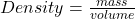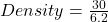## A piece of iron has a mass of 30 g and its volume is 6.2 cm3. What is its density?

Question

A piece of iron has a mass of 30 g and its volume is 6.2 cm3. What is its density?

in progress 0
7 months 2021-07-19T04:43:50+00:00 1 Answers 0 views 0

Density = 4.84 g/cm³

Explanation:

Given the following data;

Mass = 30 g

Volume = 6.2 cm³

To find the density of the piece of iron;

Density can be defined as mass all over the volume of an object.

Simply stated, density is mass per unit volume of an object.

Mathematically, density is given by the equation;Substituting into the equation, we have;Density = 4.84 g/cm³